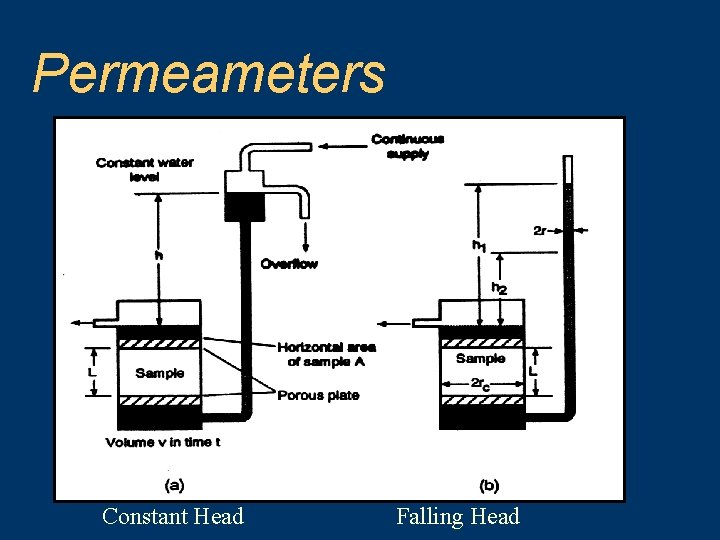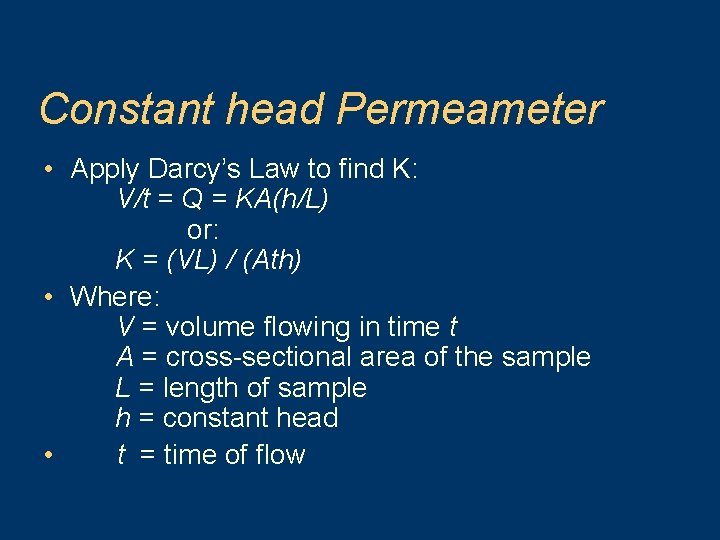# Darcys Law and Flow Philip B Bedient Civil

• Slides: 31Darcy’s Law and Flow Philip B. Bedient Civil and Environmental Engineering Rice University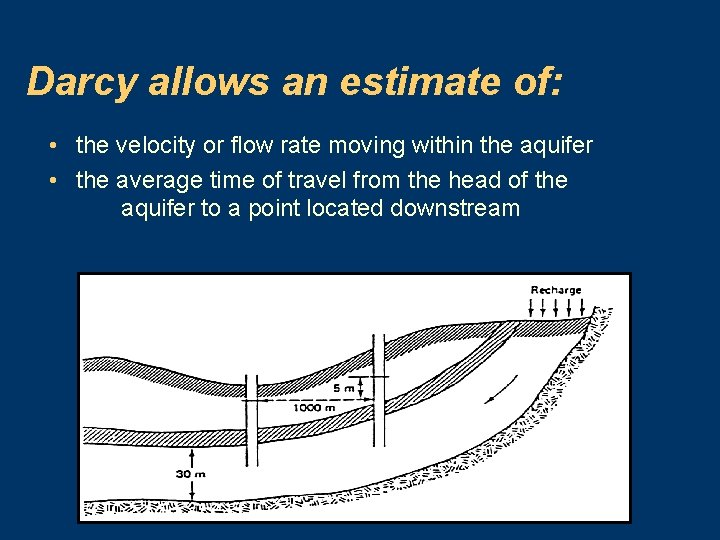Darcy allows an estimate of: • the velocity or flow rate moving within the aquifer • the average time of travel from the head of the aquifer to a point located downstreamDarcy’s Law • Darcy’s law provides an accurate description of the flow of ground water in almost all hydrogeologic environments.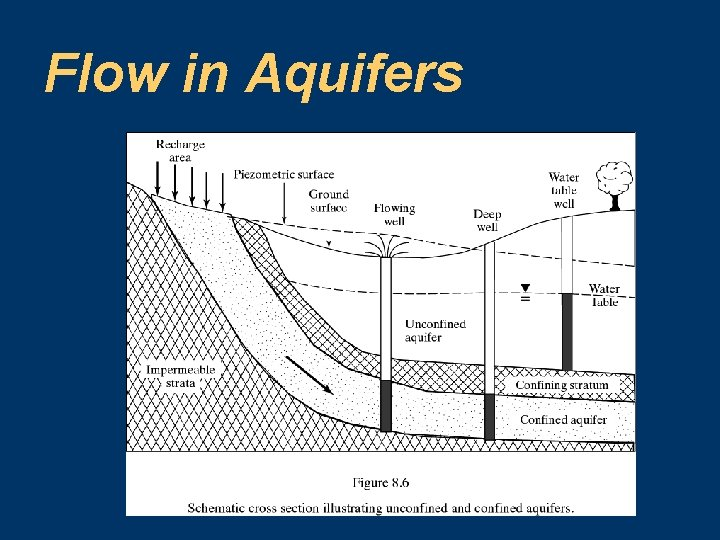Flow in AquifersDarcy’s Experiment (1856): Flow rate determined by Head loss dh = h 1 - h 2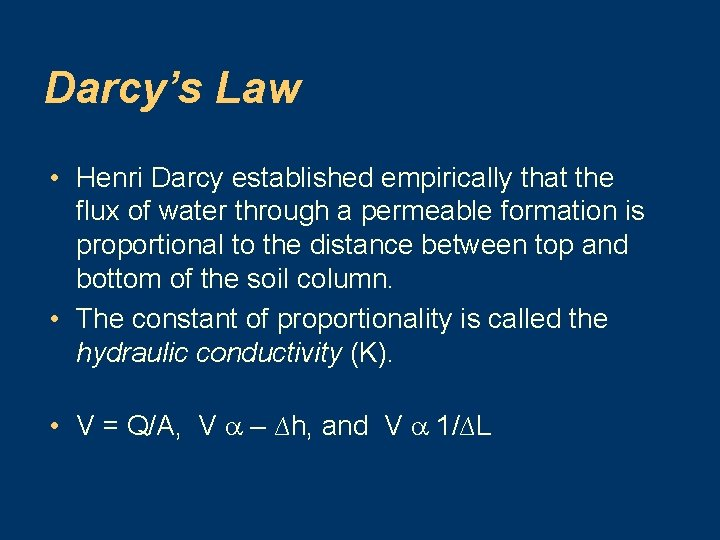Darcy’s Law • Henri Darcy established empirically that the flux of water through a permeable formation is proportional to the distance between top and bottom of the soil column. • The constant of proportionality is called the hydraulic conductivity (K). • V = Q/A, V – ∆h, and V 1/∆LDarcy’s Law V = – K (∆h/∆L) and since Q = VA (A = total area) Q = – KA (dh/d. L)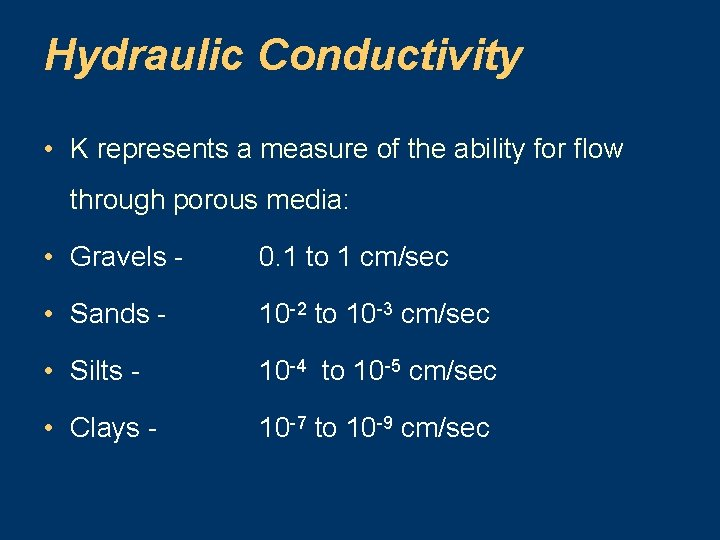Hydraulic Conductivity • K represents a measure of the ability for flow through porous media: • Gravels - 0. 1 to 1 cm/sec • Sands - 10 -2 to 10 -3 cm/sec • Silts - 10 -4 to 10 -5 cm/sec • Clays - 10 -7 to 10 -9 cm/sec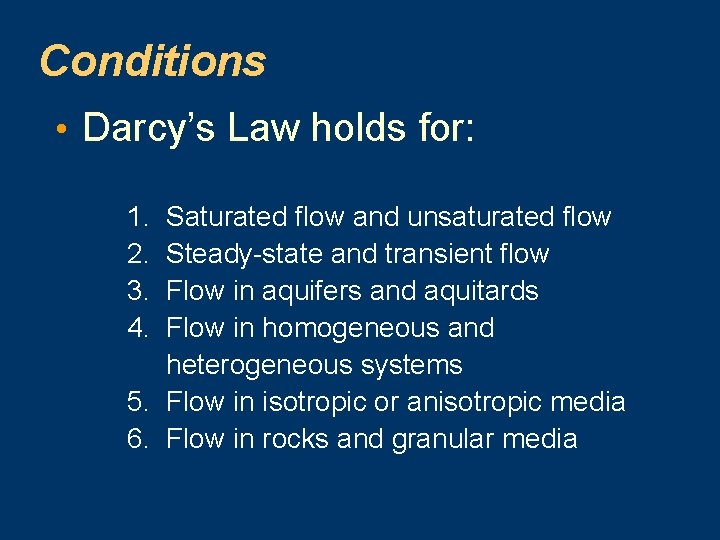Conditions • Darcy’s Law holds for: 1. Saturated flow and unsaturated flow 2. Steady-state and transient flow 3. Flow in aquifers and aquitards 4. Flow in homogeneous and heterogeneous systems 5. Flow in isotropic or anisotropic media 6. Flow in rocks and granular media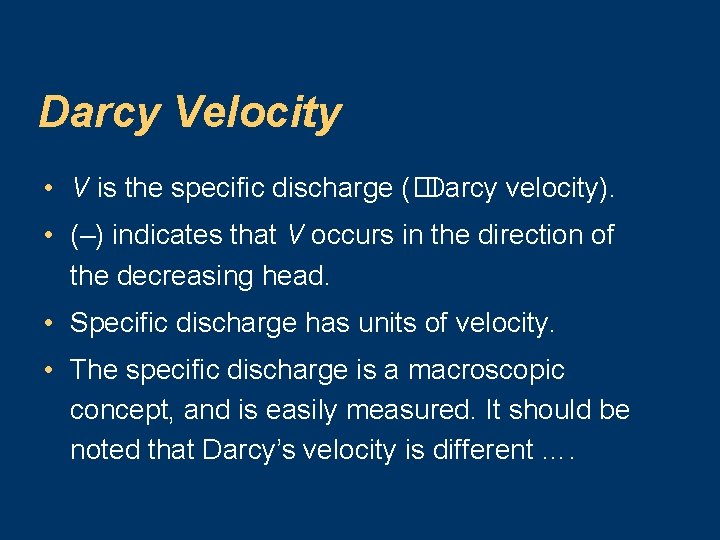Darcy Velocity • V is the specific discharge (�Darcy velocity). • (–) indicates that V occurs in the direction of the decreasing head. • Specific discharge has units of velocity. • The specific discharge is a macroscopic concept, and is easily measured. It should be noted that Darcy’s velocity is different ….Darcy Velocity • . . . from the microscopic velocities associated with the actual paths if individual particles of water as they wind their way through the grains of sand. • The microscopic velocities are real, but are probably impossible to measure.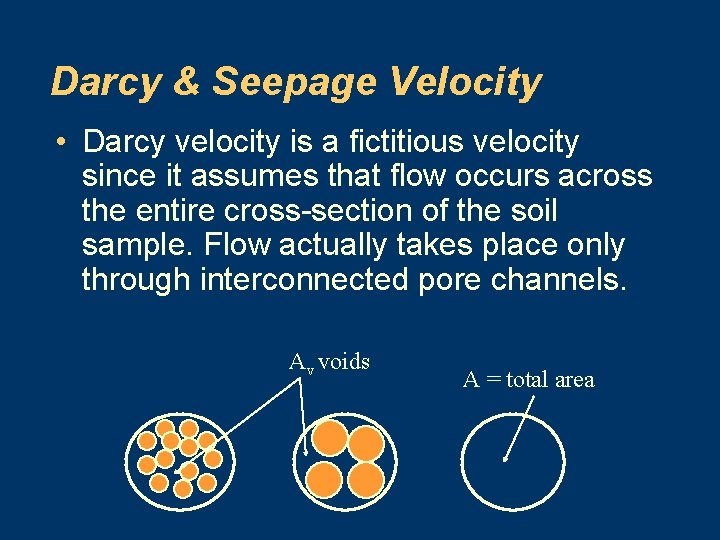Darcy & Seepage Velocity • Darcy velocity is a fictitious velocity since it assumes that flow occurs across the entire cross-section of the soil sample. Flow actually takes place only through interconnected pore channels. Av voids A = total areaDarcy & Seepage Velocity • From the Continuity Eqn: • Q = A v. D = AV Vs – Where: Q = flow rate A = total cross-sectional area of material AV = area of voids Vs = seepage velocity VD = Darcy velocityDarcy & Seepage Velocity • Therefore: VS = VD ( A/AV) • Multiplying both sides by the length of the medium (L) VS = VD ( AL / AVL ) = VD ( VT / VV ) • Where: VT = total volume VV = void volume • By Definition, Vv / VT = n, the soil porosity • Thus VS = V D / nEquations of Groundwater Flow • Description of ground water flow is based on: Darcy’s Law Continuity Equation - describes conservation of fluid mass during flow through a porous medium; results in a partial differential equation of flow. • Laplace’s Eqn - most important in math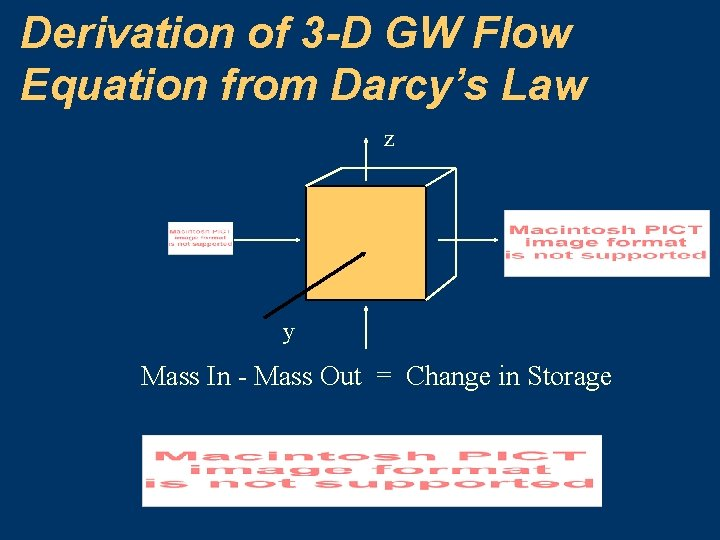Derivation of 3 -D GW Flow Equation from Darcy’s Law z y Mass In - Mass Out = Change in StorageDerivation of 3 -D GW Flow Equation from Darcy’s Law Replace Vx, Vy, and Vz with Darcy using Kx, Ky, and Kz Divide out constant , and assume Kx= Ky= Kz = KTransient Saturated Flow A change in h will produce change in and n, replaced with specific storage Ss = g( + n ). Note, is the compressibility of aquifer and B is comp of water, therefore,Solutions to GW Flow Eqns. Solutions for only a few simple problems can be obtained directly - generally need to apply numerical methods to address complex boundary conditions. h 0 h 1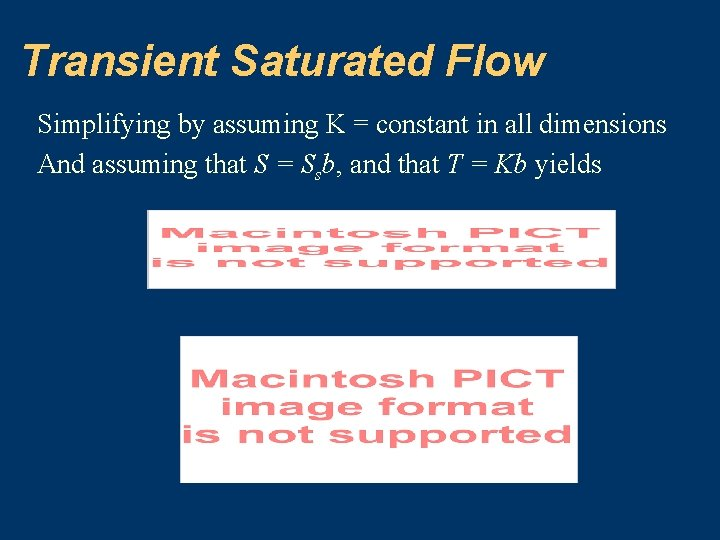Transient Saturated Flow Simplifying by assuming K = constant in all dimensions And assuming that S = Ssb, and that T = Kb yields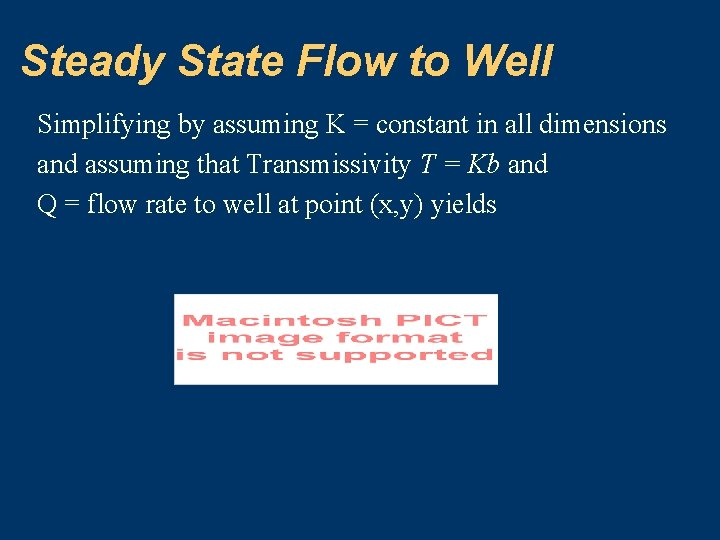Steady State Flow to Well Simplifying by assuming K = constant in all dimensions and assuming that Transmissivity T = Kb and Q = flow rate to well at point (x, y) yieldsExample of Darcy’s Law • A confined aquifer has a source of recharge. • K for the aquifer is 50 m/day, and n is 0. 2. • The piezometric head in two wells 1000 m apart is 55 m and 50 m respectively, from a common datum. • The average thickness of the aquifer is 30 m, and the average width of aquifer is 5 km.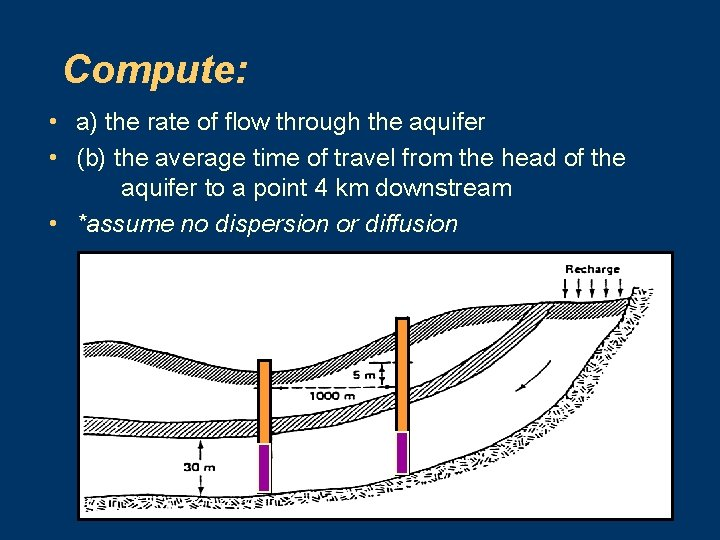Compute: • a) the rate of flow through the aquifer • (b) the average time of travel from the head of the aquifer to a point 4 km downstream • *assume no dispersion or diffusion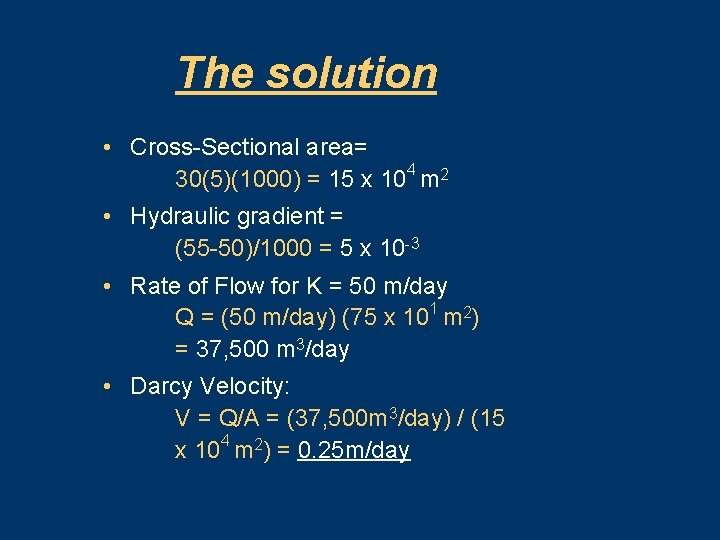The solution • Cross-Sectional area= 30(5)(1000) = 15 x 104 m 2 • Hydraulic gradient = (55 -50)/1000 = 5 x 10 -3 • Rate of Flow for K = 50 m/day Q = (50 m/day) (75 x 101 m 2) = 37, 500 m 3/day • Darcy Velocity: V = Q/A = (37, 500 m 3/day) / (15 x 104 m 2) = 0. 25 m/day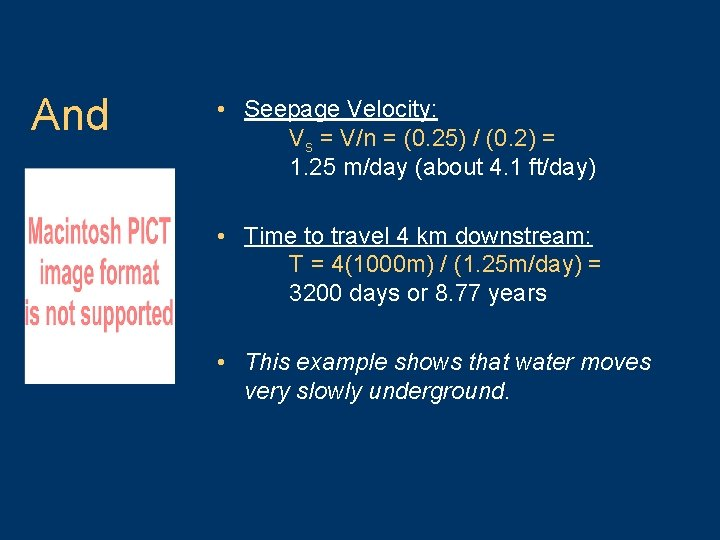And • Seepage Velocity: Vs = V/n = (0. 25) / (0. 2) = 1. 25 m/day (about 4. 1 ft/day) • Time to travel 4 km downstream: T = 4(1000 m) / (1. 25 m/day) = 3200 days or 8. 77 years • This example shows that water moves very slowly underground.Limitations of the Darcian Approach 1. For Reynold’s Number, Re, > 10 or where the flow is turbulent, as in the immediate vicinity of pumped wells. 2. Where water flows through extremely fine-grained materials (colloidal clay)Darcy’s Law: Example 2 • A channel runs almost parallel to a river, and they are 2000 ft apart. • The water level in the river is at an elevation of 120 ft and 110 ft in the channel. • A pervious formation averaging 30 ft thick and with K of 0. 25 ft/hr joins them. • Determine the rate of seepage or flow from the river to the channel.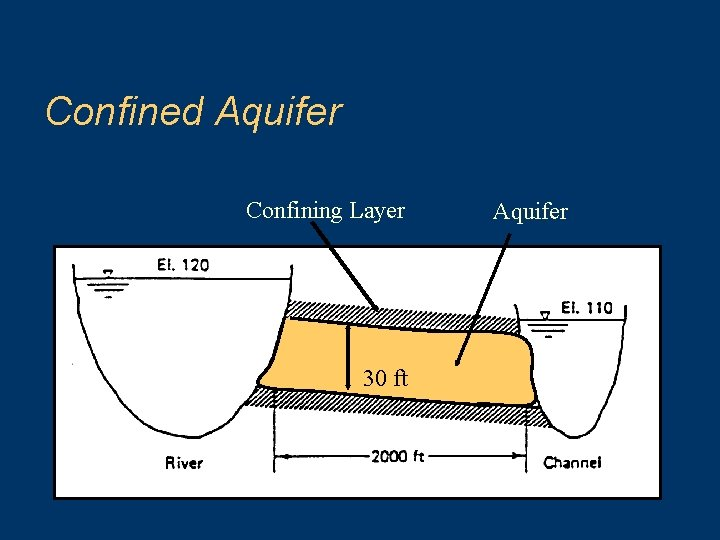Confined Aquifer Confining Layer 30 ft Aquifer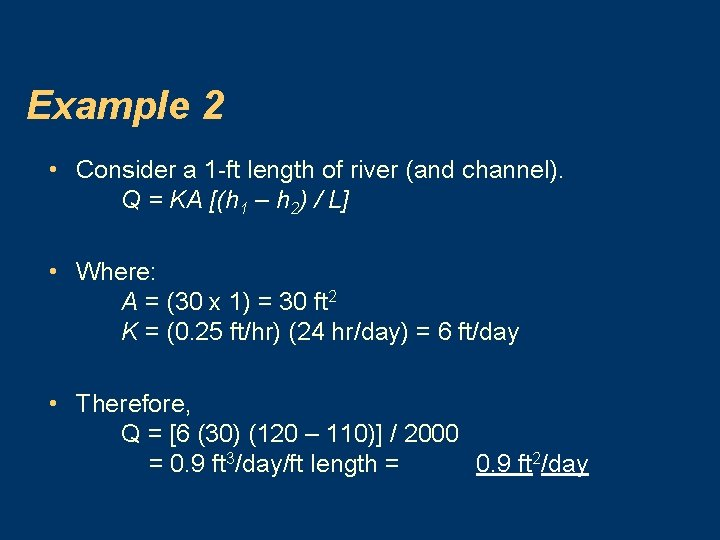Example 2 • Consider a 1 -ft length of river (and channel). Q = KA [(h 1 – h 2) / L] • Where: A = (30 x 1) = 30 ft 2 K = (0. 25 ft/hr) (24 hr/day) = 6 ft/day • Therefore, Q = [6 (30) (120 – 110)] / 2000 = 0. 9 ft 3/day/ft length = 0. 9 ft 2/day